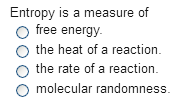Chemistry Practice Problems Entropy Practice Problems Solution: Entropy is a measure of a. free energy. b. the hea...

# Solution: Entropy is a measure of a. free energy. b. the heat of a reaction. c. the rate of a reaction. d. molecular randomness.

###### Problem

Entropy is a measure of

a. free energy.

b. the heat of a reaction.

c. the rate of a reaction.

d. molecular randomness.View Complete Written Solution

Entropy

Entropy

#### Q. Sodium reacts violently with water according to the equation: 2 Na(s) + 2 H2O(l) →2NaOH(aq) + H2(g) The resulting solution has a higher temperature th...

Solved • Mon Jul 30 2018 16:45:25 GMT-0400 (EDT)

Entropy

#### Q. Place the following in order of increasing standard molar entropy.H2O(l) H2O(g) H2O(s) a. H2O(g), H2O(s), H2O(l) b. H2O(l), H2O(s), H2O(g) c. H2O(g), ...

Solved • Mon Jul 30 2018 16:37:30 GMT-0400 (EDT)

Entropy

#### Q. Which provides the greatest decrease in entropy?

Solved • Mon Jul 30 2018 13:41:57 GMT-0400 (EDT)

Entropy

#### Q. The entropy of 1 mole of N2 (g) is greater than the entropy of 1 mole of N2(aq).a. Trueb. False

Solved • Tue Jul 24 2018 10:26:53 GMT-0400 (EDT)